Courses

# Test: Divisibility And Remainders- 2

## 15 Questions MCQ Test Additional Questions for GMAT Practice (Quant and Verbal) | Test: Divisibility And Remainders- 2

Description
This mock test of Test: Divisibility And Remainders- 2 for GMAT helps you for every GMAT entrance exam. This contains 15 Multiple Choice Questions for GMAT Test: Divisibility And Remainders- 2 (mcq) to study with solutions a complete question bank. The solved questions answers in this Test: Divisibility And Remainders- 2 quiz give you a good mix of easy questions and tough questions. GMAT students definitely take this Test: Divisibility And Remainders- 2 exercise for a better result in the exam. You can find other Test: Divisibility And Remainders- 2 extra questions, long questions & short questions for GMAT on EduRev as well by searching above.
QUESTION: 1

### When the positive integer x is divided by 9, the remainder is 5. What is the remainder when 3x is divided by 9?

Solution:

If there is a remainder of 5 when x is divided by 9, it must be true that x is five more than a multiple of 9. We can express this algebraically as x = 9a + 5, where a is a positive integer.

The question asks for the remainder when 3x is divided by 9. If x = 9a + 5, then 3x can be expressed as 3x = 27a + 15 (we just multiply the equation by 3). If we divide the right side of the equation by 9, we get 3a + 15/9.  9 will go once into 15, leaving a remainder of 6.

Alternatively, we can pick numbers. If we add the divisor (in this case 9) to the remainder (in this case 5) we get the smallest possibility for x. 9 + 5 = 14 (and note that 14/9 leaves a remainder of 5). 3x then gives us 3(14) = 42.  42/9 gives us 4 remainder 6 (since 4 × 9 = 36 and 36 + 6 = 42).

QUESTION: 2

### If (x # y) represents the remainder that results when the positive integer x is divided by the positive integer y, what is the sum of all the possible values of y such that (16 # y) = 1?

Solution:

The definition given tells us that when x is divided by y a remainder of (x # y) results.  Consequently, when 16 is divided by y a remainder of (16 # y) results.  Since (16 # y) = 1, we can conclude that when 16 is divided by y a remainder of 1 results.
Therefore, in determining the possible values of y, we must find all the integers that will divide into 16 and leave a remainder of 1.  These integers are 3 , 5, and 15.  The sum of these integers is 23.

QUESTION: 3

### If k and x are positive integers and x is divisible by 6, which of the following CANNOT be the value of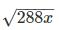?

Solution:

The valuecan be simplified to  12√2kx. Given that x is divisible by 6, for the purpose of solving this problem x might be restated as 6y, where y may be any positive integer.  The expression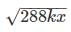could then be further simplified to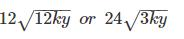Therefore each answer choice CAN be a solution if and only if there is an integer y such that 24√3ky equals that answer choice. The following table shows such an integer value of y for four of the possible answer choices, which therefore CAN be a solution.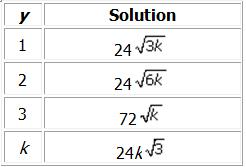The answer choice that cannot be the value ofis 24√k. For this expression to be a possible solution, y would have to equal 1/3, which is not a positive integer.  Put another way, this solution would require that x = 2, which cannot be true because x is divisible by 6.

QUESTION: 4

1025 – 560 is divisible by all of the following EXCEPT:

Solution:

First consider an easier expression such as 105 – 560. Doing the computation yields 99,440, which has 2 9's followed by 440.
From this, we can extrapolate that 1025 – 560 will have a string of 22 9's followed by 440.
Now simply apply your divisibility rules:
You might want to skip 11 first  because there is no straight forward rule for divisibility by 11. You can always return to this if necessary. [One complex way to test divisibility by 11 is to assign opposite signs to adjacent digits and then to add them to see if they add up to 0. For example, we know that 121 is divisible by 11 because -1 +2 -1 equals zero. In our case, the twenty-two 9s, when assigned opposite signs, will add up to zero, and so will the digits of 440, since +4 -4 +0 equals zero.]

If the last three digits of the number are divisible by 8, the number is divisible by 8. Since 440 is divisible by 8, the entire expression is divisible by 8.
If the last two digits of the number are divisible by 4, the number is divisible by 4. Since 40 is divisible by 4, the enter expression is divisible by 4.
If a number ends in 0 or 5, it is divisible by 5. Since the expression ends in 0, it is divisible by 5.
For a number to be divisible by three, the sum of the digits must be divisible by three. The sum of the 22 9's will be divisible by three but when you add the sum of the last three digits, 8 (4 + 4 + 0), the result will not be divisible by 3. Thus, the expression will NOT be divisible by 3.

QUESTION: 5

x, y, a, and b are positive integers. When x is divided by y, the remainder is 6. When a is divided by b, the remainder is 9. Which of the following is NOT a possible value for y + b?

Solution:

The problem states that when x is divided by y the remainder is 6.  In general, the divisor (y in this case) will always be greater than the remainder.  To illustrate this concept, let's look at a few examples:
15/4 gives 3 remainder 3 (the divisor 4 is greater than the remainder 3)
25/3 gives 8 remainder 1 (the divisor 3 is greater than the remainder 1)
46/7 gives 6 remainder 4 (the divisor 7 is greater than the remainder 4)
In the case at hand, we can therefore conclude that y must be greater than 6.
The problem also states that when a is divided by b the remainder is 9.  Therefore, we can conclude that b must be greater than 9.
If y > 6 and b > 9, then y + b > 6 + 9 > 15.  Thus, 15 cannot be the sum of y and b.

QUESTION: 6

When the integer x is divided by the integer y, the remainder is 60. Which of the following is a possible
value of the quotient x/y?
I. 15.15
II. 18.16
III. 17.17

Solution:

If the integer x divided by y has a remainder of 60, then x can be expressed as:
x = ky + 60, where k is an integer (i.e. y goes into x k times with a remainder of 60)

We could also write an expression for the quotient x/y: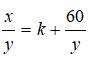Notice that k is still the number of times that y goes into x evenly.  60/y is the decimal portion of the quotient, i.e. the remainder over the divisor y.
The first step to solving this problem is realizing that k, the number of times that y goes into x evenly, can be anything for this question since we are only given a value for the remainder.  The integer values before the decimal point in answers I, II and III are irrelevant.

The decimal portion of the possible quotients in I, II and III are another story.  From the equation we have above, for a decimal to be possible, it must be something that can be expressed as 60/y, since that is the portion of the quotient that corresponds to the decimal.  But couldn't any decimal be expressed as 60 over some y?  The answer is NO because we are told in the question that y is an integer.

Let's look at answer choice I first. Is 60 / y = 0.15, where y is an integer? This question is tantamount to asking if 60 is divisible by 0.15 or if 6000 is divisible by 15? 6000 IS divisible by 15 because it is divisible by 5 (ends in a 0) and by 3 (sum of digits, 6, is divisible by 3) Therefore, answer choice I is CORRECT. Using the same logic for answer choice II, we must check to see if 6000 is divisible by 16. 6000 IS divisible by 16 because it is can be divided by 2 four times: 3000, 1500, 750, 375. Therefore, answer choice II is CORRECT. 6000 IS NOT divisible by 17 because 17 is prime and not part of the prime make-up of 6000. Therefore answer choice III is NOT CORRECT. Therefore the correct answer is D, I and II only

QUESTION: 7

The ratio of cupcakes to children at a particular birthday party is 104 to 7. Each child at the birthday party eats exactly x cupcakes (where x is a positive integer) and the adults attending the birthday party do not eat anything. If the number of cupcakes that remain uneaten is less than the number of children at the birthday party, what must be true about the number of uneaten cupcakes?
I. It is a multiple of
2. II. It is a multiple of
3. III. It is a multiple of 7.

Solution:

If the ratio of cupcakes to children is 104 to 7, we can first express the number of cupcakes and children as 104n and 7n, where n is some positive integer.  If n = 1, for example, there are 104 cupcakes and 7 children; if n = 2, there are 208 cupcakes and 14 children, etc.
We are told in the problem that each of the children eats exactly x cupcakes and that there are some number of cupcakes leftover (i.e. a remainder) that is less than the number of children.  Let’s call the remainder R.  This means that means that the number of children, 7n, goes into the number of cupcakes, 104n, x times with a remainder of R.  We can use this to write out the following equation:
104n = 7nx + R.
We are asked here to find out information about the  divisibility R.  Often times with remainder questions the easiest thing to do is to try numbers:
If n = 1, the problem becomes what is true of the remainder when you divide 104  by 7.
n = 1   104/7 = 14 remainder 6
n = 2   208/14 = 14 remainder 12
n = 3   312/21 = 14 remainder 18
Notice the pattern here.  With 104 and 7, we started out with a remainder of 6.  When we doubled both the numerator (104) and the denominator (7), the quotient remained the same (14), and the remainder (6) simply doubled.  In this particular problem, the remainder when n = 1 was 6, which as we know is divisible by 2 and 3.  Since all subsequent multiples of 104 and 7 (i.e. n = 2, 3, 4…) will yield remainders that are multiples of this original 6, the remainder will always be divisible by 2 and 3 and the answer here is D.
There is a more algebraic reason why the remainder always remains a multiple of the original remainder, 6.  Let's take for example a number x that when divided by y, qives a quotient of q with a a remainder of r.  An equation can be written: x = qy + r.  If we multiply p by some constant, c, we must multiply both sides of the equation above, i.e. xc = cqy + cr.  Notice that it is not just the x and y that get multiplied by a factor of c, but also r, the remainder!   We can generalize to say that if x divided by y has a quotient of q and a remainder of r, a multiple of x divided by that same multiple of y will have the original quotient and the same multiple of the original remainder.

QUESTION: 8

When the positive integer x is divided by 11, the quotient is y and the remainder 3. When x is divided by 19, the remainder is also 3. What is the remainder when y is divided by 19?

Solution:

If x divided by 11 has a quotient of y and a remainder of 3, x can be expressed as x = 11y + 3, where y is an integer (by definition, a quotient is an integer).  If x divided by 19 also has a remainder of 3, we can also express x as x = 19z + 3, where z is an integer.

We can set the two equations equal to each other:
11y + 3 = 19z + 3
11y = 19z

The question asks us what the remainder is when y is divided by 19. From the equation we see that 11y is a multiple of 19 because z is an integer. y itself must be a multiple of 19 since 11, the coefficient of y, is not a multiple of 19.

If y is a multiple of 19, the remainder must be zero.

QUESTION: 9

A group of n students can be divided into equal groups of 4 with 1 student left over or equal groups of 5 with 3 students left over. What is the sum of the two smallest possible values of n?

Solution:

When n is divided by 4 it has a remainder of 1, so n = 4x + 1, where x is an integer. Likewise when n is divided by 5 it has a remainder of 3, so n = 5y + 3, where y is an integer. To find the two smallest values for n, we can list possible values for n based on integer values for x and y. To be a possible value for n, the value must show up on both lists: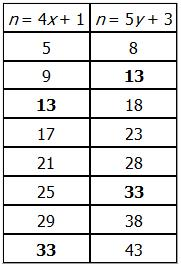The first two values for n that work with both the x and y expressions are 13 and 33. Their sum is 46.

QUESTION: 10

When x is divided by 4, the quotient is y and the remainder is 1. When x is divided by 7, the quotient is z and the remainder is 6. Which of the following is the value of y in terms of z?

Solution:

If x is divided by 4 and has a quotient of y and a remainder of 1, then x = 4y + 1.
And if x divided by 7 and has a quotient of z and a remainder of 6, then x = 7z + 6.
If we combine these two equations, we get:
4y + 1 = 7z + 6
4y = 7z + 5, so we have y = (7z + 5) / 4.
You could also solve this problem by picking a value for x. The trick is to pick a value that works with the constraints given in the problem.
One such value is x = 13. This means that y is equal to the quotient of x ÷ 4, which is 3. The remainder would be 1, which meets the constraint given in the problem.
Given that x = 13, z is equal to the quotient of x ÷ 7, which is 1. The remainder would be 6, which meets the constraint given in the problem.
Thus x = 13, y = 3, and z = 1 meet the constraints given in the problem. Plug the value z = 1 into each answer choice to see which choice yields the correct value for y, which is 3. Only answer choice D works.

QUESTION: 11

If n is an integer and n4 is divisible by 32, which of the following could be the remainder when n is divided by 32?

Solution:

The prime factors of n4 are really four sets of the prime factors of the integer n.

Since n4 is divisible by 32 (or 25), n4 must be divisible by 2 at least 5 times. What does this tell us about the integer n?

If n is divisible by only one 2, then n4 would be divisible by exactly four 2's (since the prime factors of n4 have no source other than the integer n).

But we know that n4 is divisible by at least five 2's! This means that n must be divisible by at least two 2's (which means that n4 must be divisible by eight 2's). Thus, we know that the integer n must be divisible by 4.

Now that we know that n is divisible by 4, we can consider what happens when we divide n by 32.
If we divide n by 32 we can represent this mathematically as follows:
n = 32b + c   (where b is the number of times 32 goes into n and c is the integer remainder)
We know that n is divisible by 4 so we can rewrite this as:

4x = 32b + c               (where x is an integer)
This equation can be simplified, by dividing both sides by 4 as follows:

x = 8b + c/4

Since we know that x is an integer, the sum of 8b and c/4 must yield an integer. We know that 8b is an integer so c/4 must be also be an integer. Therefore, c, the remainder, must be divisible by 4.
Only answer choice B qualifies. The remainder when n is divided by 32 could be 4. It could not be any of the another answer choices. The correct answer is B.

QUESTION: 12

x1 and x2 are each positive integers. When x1 is divided by 3, the remainder is 1, and when x2 is divided by 12, the remainder is 4. If y = 2x1 + x2, then what must be true about y?
I. y is even II. y is odd III. y is divisible by 3

Solution:

The statement “when x1 is divided by 3, the remainder is 1” can be translated mathematically as follows:  There exists an integer n such that x1 =3n+1
Similarly, the statement "when xis divided by 12, the remainder is 4" can be translated mathematically as follows: There exists an integer m such that
x2=12m+4.
=y=2x1+x2
=2(3n+1+(12m+4)
= 6n+2+12m+4
Therefore:
=6(n+2m+1)
The expression (n + 2m + 1) must be an integer (since n and m are both integers). Therefore, y is equal to some integer multiplied by 6. This means that y is divisible by 6. Any number that is divisible by 6 must also be divisible by both 2 and 3. Hence, y must be both even and divisible by 3. Consequently, I and III must be true and the correct answer is D.

QUESTION: 13

The sum of all the digits of the positive integer q is equal to the three-digit number x13. If q = 10n – 49, what is the value of n?

Solution:

We are first told that the sum of all the digits of the number q is equal to the three-digit number x13. Then we are told that the number q itself is equal to 10n - 49. Finally, we are asked for the value of n.

The first step is to recognize that  10n - 49 will have to equal a series of 9's ending with a 5 and a 1 (99951, for example, is 105 - 49 ). So q is a series of 9's ending with a 5 and a 1. Since the sum of all the digits of q is equal to x13, we know that x13 is the sum of all those 9's plus 5 plus 1. So if we subtract 5 and 1 from x13, we are left with x07.

This three-digit number x07 is the sum of all the 9's alone. So x07 must be a multiple of 9. For any multiple of 9, the sum of all the digits of that multiple must itself be a multiple of 9 (for example, 585 = (9)(65) and 5 + 8 + 5 = 18, which is a multiple of 9). So it must be true that x + 0 + 7 is a multiple of 9. The only single-digit value for x that will yield a multiple of 9 when added to 0 and 7 is 2. Therefore, x = 2 and the sum of all the 9's in q is 207.

Since 207 is a multiple of 9, we can set up the equation 9y = 207, where y is a positive integer.  Solving for y, we get y = 23. So we know q consists of a series of twenty-three 9's followed by a 5 and a 1: 9999999999999999999999951. If we add 49 to this number, we get 10,000,000,000,000,000,000,000,000.

Since the exponent in every power of 10 represents the number of zeroes (e.g.,102 = 100  , which has two zeroes;  103=1000 which has three zeroes, etc.), we must be dealing with  1025. Thus n = 25.

QUESTION: 14

If positive integer n is divisible by both 4 and 21, then n must be divisible by which of the following?

Solution:

If n is divisible by both 4 and 21, its prime factors include 2, 2, 3, and 7. Therefore, any integer that can be constructed as the product of these prime factors is also a factor of n. In this case, 12 is the only integer that can definitively be constructed from the prime factors of n, since 12 = 2 x 2 x 3.

QUESTION: 15

Susie can buy apples from two stores: a supermarket that sells apples only in bundles of 4, and a
convenience store that sells single, unbundled apples. If Susie wants to ensure that the total number of
apples she buys is a multiple of 5, what is the minimum number of apples she must buy from the
convenience store?

Solution:

Since the supermarket sells apples in bundles of 4, we can represent the number of apples that Susie buys from the supermarket as 4x, where x can be any integer ≥ 0. If the number of apples that Susie buys from the convenience store is simply y, the total number of apples she buys is (4x + y). We are asked to find the smallest possible value of y such that (4x + y) can be a multiple of 5.

We can solve this problem by testing numbers. Since the question asks us what is the minimum value for y such that (4x + y) can be a multiple of 5, it makes sense to begin by testing the smallest of the given answer choices. If y=0, can (4x + y) be a multiple of 5? Yes, because x could equal 5. (The value of (4(5) + 0) is 20, which is a multiple of 5.)